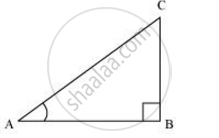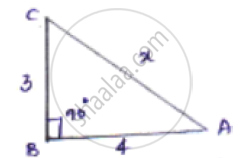Share
Notifications

View all notifications
Books Shortlist
Your shortlist is empty

# If 3 cot A = 4, Check whether ((1-tan^2 A)/(1+tan^2 A)) = cos^2 A - sin^2 A or not - CBSE Class 10 - Mathematics

Login
Create free account

Forgot password?
ConceptTrigonometric Ratios of an Acute Angle of a Right-angled Triangle

#### Question

If 3 cot A = 4, Check whether ((1-tan^2 A)/(1+tan^2 A)) = cos^2 A - sin^2 A or not

#### Solution 1

It is given that 3cot A = 4 or  cot A = 4/3

Consider a right triangle ABC, right-angled at point B.cot A = ("Side adjacent to" angle A)/("Side opposite to"angle A)

(AB)/(BC) =  4/3

If AB is 4k, then BC will be 3k, where k is a positive integer.

In ΔABC,

(AC)2 = (AB)2 + (BC)2

= (4k)2 + (3k)2

= 16k2 + 9k2

= 25k2

AC = 5k

cos A = ("Side adjacent to"angleA)/"Hypotenuse" = (AB)/(AC)

= 4k/5k = 4/5

sin A = ("Side adjacent to"angleA)/"Hypotenuse" = (BC)/(AC)

=3k/5k = 3/5

tan A = ("Side adjacent to"angleA)/"Hypotenuse" = (BC)/(AB)

= 3k/4k = 3/4

(1-tan^2 A)/(1+tan^2 A) = ((1 - (3/4)^2)/(1+(3/4)^2)) = ((1-9/16)/(1+9/16))

= (7/16)/(25/16) =  7/25

cos2 A + sin2 A = (4/5)2 - (3/5)2

= 16/25 - 9/25 = 7/25

:. (1-tan^2A)/(1+tan^2 A)= cos^2A - sin^2A

#### Solution 2

3 cot A = 4, check = (1 - tan^2 A)/(1 + tan^2 A) = cos^2 A - sin^2 Acot A = "𝑎𝑑𝑗𝑎𝑐𝑒𝑛𝑡 𝑠𝑖𝑑𝑒"/"𝑜𝑝𝑝𝑜𝑠𝑖𝑡𝑒 𝑠𝑖𝑑𝑒" = 4/3

Let x be the hypotenuse

By Applying Pythagoras theorem

𝐴𝐶2 = 𝐴𝐵2 + 𝐵𝐶2

𝑥2 = 42 + 32

𝑥2 = 25

𝑥 = 5

Tan A = 1/(cos^2 A) = 3/4

cos A = "𝑎𝑑𝑗𝑎𝑐𝑒𝑛𝑡 𝑠𝑖𝑑𝑒"/"ℎ𝑦𝑝𝑜𝑡𝑒𝑛𝑢𝑠𝑒" = 4/5

sin A = 3/5

L.H.S = (1 - tan^2 A)/(1 + tan^2 A)= (1 - (3/4)^2)/(1 + (3/4)^2) = ((16 - 9)/16)/((16 + 9)/16) = 7/25

R.H.S cos^2A - sin^2 A = (4/5)^2 - (3/5)^2 = (16 - 9)/25

= 7/25

Is there an error in this question or solution?

#### APPEARS IN

NCERT Solution for Mathematics Textbook for Class 10 (2019 to Current)
Chapter 8: Introduction to Trigonometry
Ex.8.10 | Q: 8 | Page no. 181
Solution If 3 cot A = 4, Check whether ((1-tan^2 A)/(1+tan^2 A)) = cos^2 A - sin^2 A or not Concept: Trigonometric Ratios of an Acute Angle of a Right-angled Triangle.
S